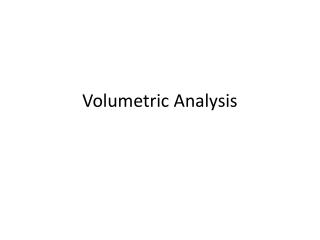# Volumetric Analysis - PowerPoint PPT PresentationDownload PresentationVolumetric Analysis

Volumetric Analysis
Download Presentation## Volumetric Analysis

- - - - - - - - - - - - - - - - - - - - - - - - - - - E N D - - - - - - - - - - - - - - - - - - - - - - - - - - -
##### Presentation Transcript

1. Volumetric Analysis

2. Volumetric Analysis • Volumetric analysis involves the analysis of a solution of unknown concentration with a standard solution. • A pipette is used to transfer a known quantity of one of the solutions into a conical flask. This sample is called an aliquot • The other solution is added to the burette. The amount of this solution that is needed to react with the aliquot is called the titre.

3. Volumetric Analysis (cont.) • The point where the reactants are in exact mole ratio proportion is called the equivalence point. • The point where we detect the change through an indicator or pH meter is called the end point. • Titrations are repeated until three titrations are concordant, i.e. within 0.1ml of each other

4. Standard Solutions – Primary Standard • A standard solution is a solution of precisely known concentration. For a solution to be used as a primary standard it must • be readily obtainable in a pure form • have a known formula • be easy to store without deteriorating or reacting with the atmosphere • have a high molar mass to minimise the effect of errors in weighing • be inexpensive.

5. Standard Solutions – Secondary standards • If a primary standard solution can not be made a secondary standard solution is created by titrating it with a primary standard to find the exact concentration. • Substances such as strong acids and strong bases are not suitable for primary standards as the concentrations prepared are not accurate and they can react with the atmosphere or containers • Examples of recommended substances used to make secondary standards can be found in the table on the next slide

6. Flow Chart for a Titration

7. Back Titrations • If an end point cannot be easily detected in a titration or the substance is toxic, volatile, a mixture of gases, in low concentration or insoluble in water a back titration is needed. • A back titration involves first reacting the sample with an excess amount of a standard solution and titrating the product or excess with another suitable standard solution. • Flow charts of back titrations can be found on the following slides

8. Product Back tTtration

9. Excess Reactant Back Titration

10. Acid-Base Titrations • When completing an acid-base titration it is important to select an appropriate indicator to determine the end point. • The pH of the equivalence point varies depending on the reactants that are used. • The following slides show the pH curves of different acid and base reactions.

11. Strong Acid – Strong Base

12. Strong Acid – Weak Base

13. Weak Acid – Strong Base

14. Weak Acid – Weak Base (unsuitable for titration)

15. Choosing an appropriate indicator • When selecting an indicator it is necessary to find one that changes colour in the range that has the sharp change in pH.

16. Redox Reactions • Redox reactions occur when reactant loses reactions (oxidation) and another reactant gains electrons (reduction). • These reactions occur spontaneously in solution and usually result in a change in colour, which can be used to find the end point.

17. Oxidation Number Rules Revision • All free elements have an oxidation number of 0 • Ionic compounds containing two ions have oxidation numbers equal to their ions (related to their group number) • Oxygen is 2- except in peroxides (H2O2, BaO2) where it is 1- • Hydrogen is 1+ except in metal hydrides (NaH, CaH2) where it is 1- • The sum of oxidation numbers in a neutral compound is 0 • The sum of oxidation numbers in a polyatomic ion is equal to the charge on the ion. • The most electronegative element has the negative oxidation number